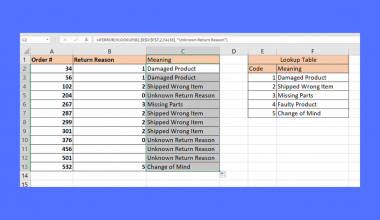# How to Sort IP Address from Low to High in Excel

This guide will explain in detail how you can sort IP addresses from low to high in Microsoft Excel.

Since IP addresses usually omit leading zeros, using the built-in sorting techniques may lead to an inaccurate sort.

To understand why sorting IP addresses is not a straightforward task, we must first look into how an IP address is structured.

An IP address is a 32-bit number that is formatted as four 8-bit fields separated by periods. Let’s consider the following 32-bit number:

`11000000.10011110.00000001.00100110`

Every byte is a sequence of eight bits, each separated by a period. If we were to convert each byte into decimal, we would have the following:

`192.158.1.38`

But what happens if we try to compare this value with another IP address like 192.158.1.102

If we used the alphabetical sort, the value ending with 102 would come before the value ending in 38. This is an incorrect sort since the former is actually of a higher value than the latter.

To remedy this, we will have to add leading zeros into our IP addresses when comparing them. In the example below, you can observe that adding zeros to the start of each byte leads to the correct type of sort.Now that we know how to correctly sort an IP address, is it possible to perform this solution in Excel?

In the next section we will explore an actual spreadsheet that solves this IP address sorting issue. We will then explain the formulas and methods used in that spreadsheet.

## A Real Example of Sorting IP Addresses in Excel

Let’s take a look at an actual spreadsheet that successfully sorts IP addresses by extending the bytes with leading zeroes.

The sample spreadsheet below has successfully sorted the IP addresses provided in Column A. The table used a formula to extend the IP address with leading zeroes. Each byte should now have three digits.To get the values in Column C, we just need to use the following lengthy formula:

`=TEXT(LEFT(A3;FIND(".";A3;1)-1);"000") & "." & TEXT(MID(A3;FIND( ".";A3;1)+1;FIND(".";A3;FIND(".";A3;1)+1)-FIND(".";A3;1)-1);"000") & "." & TEXT(MID(A3;FIND(".";A3;FIND(".";A3;1)+1)+1;FIND(".";A3; FIND(".";A3;FIND(".";A3;1)+1)+1)-FIND(".";A3;FIND(".";A3;1)+1)-1); "000") & "." & TEXT(RIGHT(A3;LEN(A3)-FIND(".";A3;FIND(".";A3;FIND( ".";A3;1)+1)+1));"000")`

The formula above consists of four concatenations. Let’s focus on the first string being concatenated:

`TEXT(LEFT(A3;FIND(".";A3;1)-1);"000") `

The `LEFT(A3;FIND(".";A3;1)-1)` simply returns the byte found before the first period. We then use the `TEXT` formula to format the number to add leading zeros. The other three instances of the `TEXT` function in the main formula do a similar procedure.

You can make your own copy of the spreadsheet above using the link attached below.

If you’re ready to try sorting IP addresses yourself in Excel, let’s begin writing it ourselves! Head over to the next section to learn how.

## How to Sort IP Address from Low to High in Excel

This section will guide you through the steps needed to sort IP addresses from low to high in Excel accurately. You’ll learn how we can use a formula with `TEXT`, `LEFT`, and `FIND` functions to add leading zeros to our original IP addresses.

Follow these steps to start using the IP address formula:

1. First, select the cell that will hold the formula for expanding IP addresses. In this example, we’ve chosen cell B2 directly to the right of the first IP address to sort.2. Next, we can paste our IP address formula into the formula bar. Ensure that the cell being referenced lines up with the location of the IP address you want to expand.3. Simply drag down the formula in B2 to fill out the rest of column B. Each IP address should now have a total of twelve digits each.4. Next, we can paste the values in column B to another cell range. We use the Paste Special option that returns the values rather than the original formula.5. Select the newly pasted data. Head to the Data tab and find the A-Z sort icon. This sorting order will sort the values from lowest to the highest value.6. Excel will ask the user if they want to expand the selection during the sort. We want to select this option so that the values in column A will also follow the sort.7. The original IP address values in Excel should now be sorted in the correct order from low to high.That’s all you need to remember to start sorting IP addresses  in Excel. This step-by-step guide shows how we can use the `LEFT`, `TEXT`, and `FIND` functions to add leading zeros to each of the four bytes that make up an IP address.

Sorting IP addresses is just one way you can use Excel to perform numerical tasks with your data. With so many other Excel functions out there, you can surely create the perfect formulas for your spreadsheet.

Are you interested in learning more about what Excel can do? Make sure to subscribe to our newsletter to be the first to know about the latest guides and tutorials from us.### Get emails from us about Excel.

Our goal this year is to create lots of rich, bite-sized tutorials for Excel users like you. If you liked this one, you'd love what we are working on! Readers receive ✨ early access ✨ to new content.

##### You May Also Like## How to Use ISERROR with VLOOKUP in Excel

This guide will explain how you can use the ISERROR function in Excel to catch and handle errors…## How to Fix Formula Not Showing Correct Result in Excel

This tutorial will explain a few ways to fix formulas not showing the correct result in Excel. Microsoft…## How to Merge Two Files in Excel

This guide will explain several methods you can use to merge two files in Excel. When working with…## How to Use a Data Model in Excel

This guide will explain how to start using a data model in Excel. Excel’s Data Model tool is…